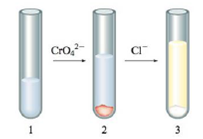Chapter 16, Problem 5ALQ

Chapter
Section
Textbook Problem

# Explain the following phenomenon: You have a test tube with an aqueous solution of silver nitrate as shown in test tube l below. A few drops of aqueous sodium chromate solution was added with the end result shown in test tube 2. A few drops of aqueous sodium chloride solution was then added with the end result shown in test tube 3.Use the Ksp values in the book to support your explanation, and include the balanced equations. Also, list the ions that are present in solution in each test tube.

Interpretation Introduction

Interpretation:

The following phenomenon is to be explained. The balanced chemical equation for each reaction is to be stated. Also, the ions that are present in solution in each test tube are to be named.

Concept introduction:

When substances having positive or negative charges react with ionic solutions to remove some ions selectively by replacing them with ions of other kinds, such reactions are called as ion-exchange reactions. The solubility of a solid is lowered if the solution already contains common ions to the solid. The chemical equation is said to be balanced when the number of atoms of chemical species on the reactant side is equal to the number of chemical species on the product side.

Explanation

Explanation

To determine: The given phenomenon with the balanced chemical equation for each reaction and the ions that are present in solution in each test tube.

The test tube 1 contains aqueous solution of AgNO3 gives Ag+ and NO3 ions. The chemical equation is,

AgNO3(s)Ag+(aq)+NO3(aq)

The test tube 1 contains Ag+ and NO3 ions.

When sodium chromate solution is added in test tube 1 then CrO42 reacts with Ag+ ions to form silver chromate precipitate. The net ionic equation is,

2Ag+(aq)+CrO42(aq)Ag2CrO4(s)

The solubility product (Ksp) value of Ag2CrO4 is 9

### Still sussing out bartleby?

Check out a sample textbook solution.

See a sample solution

#### The Solution to Your Study Problems

Bartleby provides explanations to thousands of textbook problems written by our experts, many with advanced degrees!

Get Started